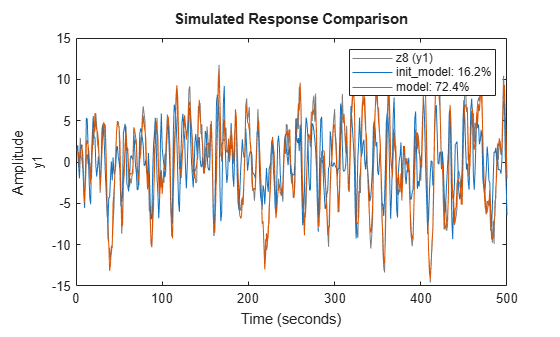Documentation

## Refine ARMAX Model with Initial Parameter Guesses at Command Line

This example shows how to refine models for which you have initial parameter guesses.

Estimate an ARMAX model for the data by initializing the A , B , and C polynomials. You must first create a model object and set the initial parameter values in the model properties. Next, you provide this initial model as input to `armax` , `polyest` , or `pem` , which refine the initial parameter guesses using the data.

`load iddata8`

Define model parameters.

Leading zeros in B indicate input delay (nk), which is 1 for each input channel.

```A = [1 -1.2 0.7]; B{1} = [0 1 0.5 0.1]; % first input B{2} = [0 1.5 -0.5]; % second input B{3} = [0 -0.1 0.5 -0.1]; % third input C = [1 0 0 0 0]; Ts = 1;```

Create model object.

`init_model = idpoly(A,B,C,'Ts',1);`

Use polyest to update the parameters of the initial model.

`model = polyest(z8,init_model);`

Compare the two models.

`compare(z8,init_model,model)`